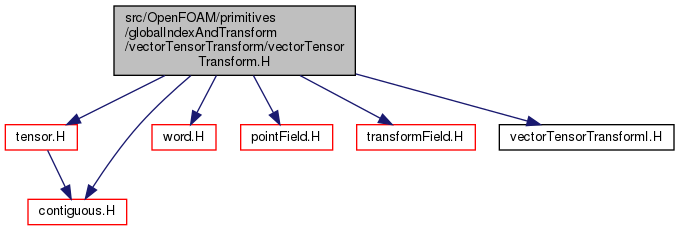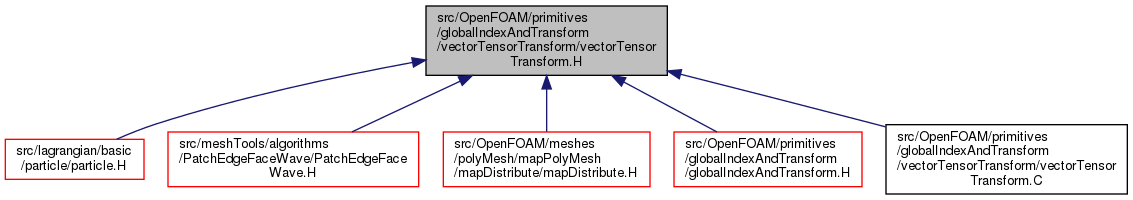The OpenFOAM Foundation
vectorTensorTransform.H File Reference
Include dependency graph for vectorTensorTransform.H:This graph shows which files directly or indirectly include this file:Go to the source code of this file.

## Classes

class  vectorTensorTransform
Vector-tensor class used to perform translations and rotations in 3D space. More...

## Namespaces

Foam
Namespace for OpenFOAM.

## Functions

Istream & operator>> (Istream &is, vectorTensorTransform &)

Ostream & operator<< (Ostream &os, const vectorTensorTransform &C)

vectorTensorTransform inv (const vectorTensorTransform &tr)
Return the inverse of the given vectorTensorTransform. More...

word name (const vectorTensorTransform &)
Return a string representation of a vectorTensorTransform. More...

template<>
bool contiguous< vectorTensorTransform > ()
Data associated with vectorTensorTransform type are contiguous. More...

bool operator== (const vectorTensorTransform &tr1, const vectorTensorTransform &tr2)

bool operator!= (const vectorTensorTransform &tr1, const vectorTensorTransform &tr2)

vectorTensorTransform operator+ (const vectorTensorTransform &tr, const vector &t)

vectorTensorTransform operator+ (const vector &t, const vectorTensorTransform &tr)

vectorTensorTransform operator- (const vectorTensorTransform &tr, const vector &t)

vectorTensorTransform operator & (const vectorTensorTransform &tr1, const vectorTensorTransform &tr2)

## Detailed Description

Original source file vectorTensorTransform.H

Definition in file vectorTensorTransform.H.